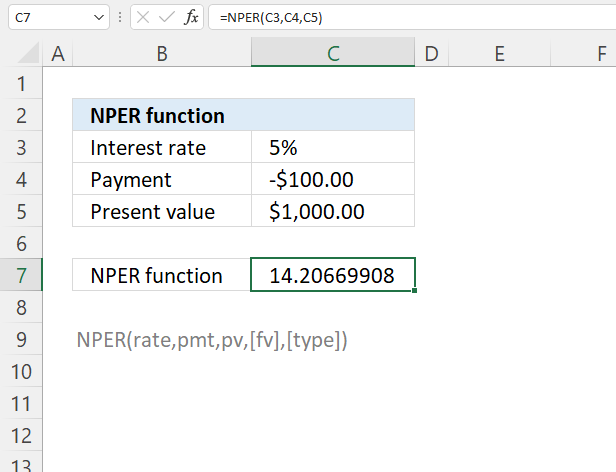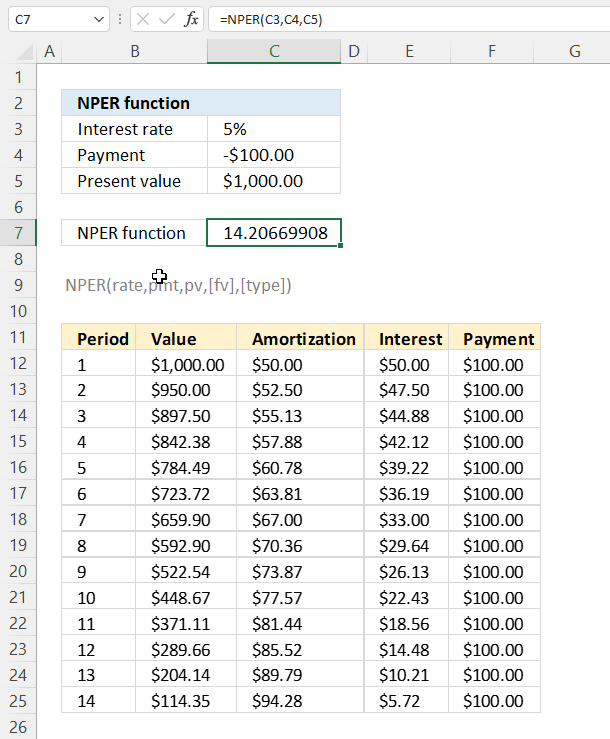Author: Oscar Cronquist Article last updated on June 28, 2022The NPER function calculates the number of periods for an investment based on periodic, constant payments and a fixed interest rate.

## 1. NPER Function Syntax

NPER(rate, pmt, pv, [fv], [type])

## 2. NPER Function Arguments

 rate Required. The interest rate per period. pmt Required. The payment per period; it must be constant. pv Required. Argument pv is an abbreviation for present value, in other words, the total value right now based on future payments. [fv] Optional. The future value, or the value you want to achieve when the last payment is made. Default value is 0 (zero). [type] Optional.  When payments are due. 0 (zero) - Default value. At the end of the period. 1 - At the beginning of the period.

## 3. NPER Function ExampleThis example shown in the image above demonstrates the NPER function in cell C7. It calculates the number of periods needed to repay \$1000 based on a constant payment of \$100 and a fixed interest rate of 5%.

Formula in cell F3:

=NPER(C3,C4,C5)

The table below the calculation shows interest, amortization, and the remaining loan amount after each periodic payment. Get the workbook to see how the calculations are made, link below.